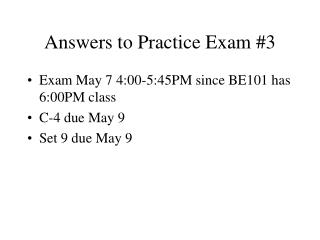# Answers to Practice Exam #3 - PowerPoint PPT PresentationDownload PresentationDownload Presentation## Answers to Practice Exam #3

- - - - - - - - - - - - - - - - - - - - - - - - - - - E N D - - - - - - - - - - - - - - - - - - - - - - - - - - -
##### Presentation Transcript

1. Answers to Practice Exam #3 • Exam May 7 4:00-5:45PM since BE101 has 6:00PM class • C-4 due May 9 • Set 9 due May 9

2. 1.PERT

5. 1C: • CRITICAL PATH VARIANCE= SUM OF VARIANCES ON CP= VARIANCES OF A,C,E,F = 4+.6+.5+.1=5.2 =5.2 • STANDARD DEVIATION = SQUARE ROOT OF 5.2 = 2.28

6. NORMAL TABLE • Z=(27-24)/2.28 = 1.32 • ROW 1.3 • COL 0.02 = .02 • CUMULATIVE PROB = .5 + .4066 = .9066 FROM TAYLOR, P 738

7. 2. SHORTEST ROUTE